1561691263

# PyTorch Tutorial for Beginners

## Introduction

Today deep learning is going viral and is applied to a variety of machine learning problems such as image recognition, speech recognition, machine translation, and others. There is a wide range of highly customizable neural network architectures, which can suit almost any problem when given enough data. Each neural network should be elaborated to suit the given problem well enough. You have to fine tune the hyperparameters of the network (the learning rate, dropout coefficients, weight decay, and many others) as well as the number of hidden layers, and the number of units in each layer. Choosing the right activation function for each layer is also crucial and may have a significant impact on metric scores and the training speed of the model.

## Activation Functions

The activation function is an essential building block for every neural network. We can choose from a huge list of popular activation functions from popular Deep Learning frameworks, like ReLU, Sigmoid, Tanh, and many others.

However, to create a state of the art model, customized particularly for your task, you may need to use a custom activation function, which is absent in Deep Learning framework you are using. Activation functions can be roughly classified into the following groups by complexity:

1. Simple activation functions like SiLU, Inverse Square Root Unit (ISRU). You can quickly implement these functions using any Deep Learning framework.
2. Activation functions with trainable parameters like Soft Exponentialactivation or S-shaped Rectified Linear Unit (SReLU).
3. Activation functions, which are not differentiable at some points and require the custom implementation of the backward step, for example, Bipolar Rectified Linear Unit (BReLU).

In this tutorial, I cover the implementation and demo examples for all of these types of functions with PyTorch framework. You can find all the code for this article on GitHub.

## Setting Up

To go through the examples of the implementation of activation functions, you would require:

• To install PyTorch,
``````# Import basic libraries
import numpy as np # linear algebra
import pandas as pd # data processing, CSV file I/O (e.g. pd.read_csv)
from collections import OrderedDict

# Import PyTorch
import torch # import main library
import torch.nn as nn # import modules
from torch.autograd import Function # import Function to create custom activations
from torch.nn.parameter import Parameter # import Parameter to create custom activations with learnable parameters
from torch import optim # import optimizers for demonstrations
import torch.nn.functional as F # import torch functions
from torchvision import datasets, transforms # import transformations to use for demo

``````

The necessary imports

``````  # Define a transform
transform = transforms.Compose([transforms.ToTensor()])

``````

Prepare the dataset

The last thing is to set up a sample function, which runs the model training process and prints out the training loss for each epoch:

``````# helper function to train a model
'''
Function trains the model and prints out the training log.
INPUT:
model - initialized PyTorch model ready for training.
'''
#setup training

#define loss function
criterion = nn.NLLLoss()
#define learning rate
learning_rate = 0.003
#define number of epochs
epochs = 5
#initialize optimizer

#run training and print out the loss to make sure that we are actually fitting to the training set
print('Training the model. Make sure that loss decreases after each epoch.\n')
for e in range(epochs):
running_loss = 0
images = images.view(images.shape, -1)
log_ps = model(images)
loss = criterion(log_ps, labels)

loss.backward()
optimizer.step()

running_loss += loss.item()
else:
# print out the loss to make sure it is decreasing
print(f"Training loss: {running_loss}")

``````

A sample model training function

Now everything is ready for the creation of models with custom activation functions.

## Implementing Simple Activation Functions

The most simple common activation functions

• are differentiable and don’t need the manual implementation of the backward step,
• don’t have any trainable parameters. All their parameters should be set in advance.

One of the examples of such simple functions is Sigmoid Linear Unit or just SiLU, also known as Swish-1: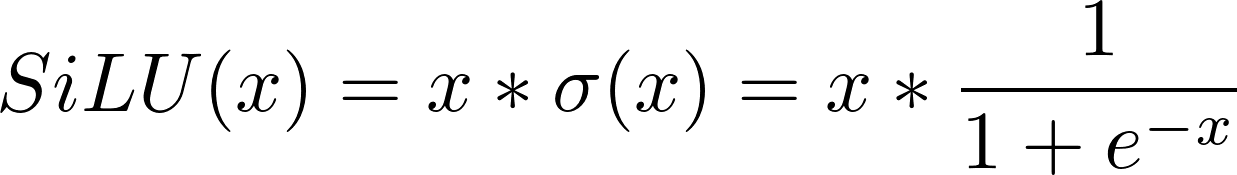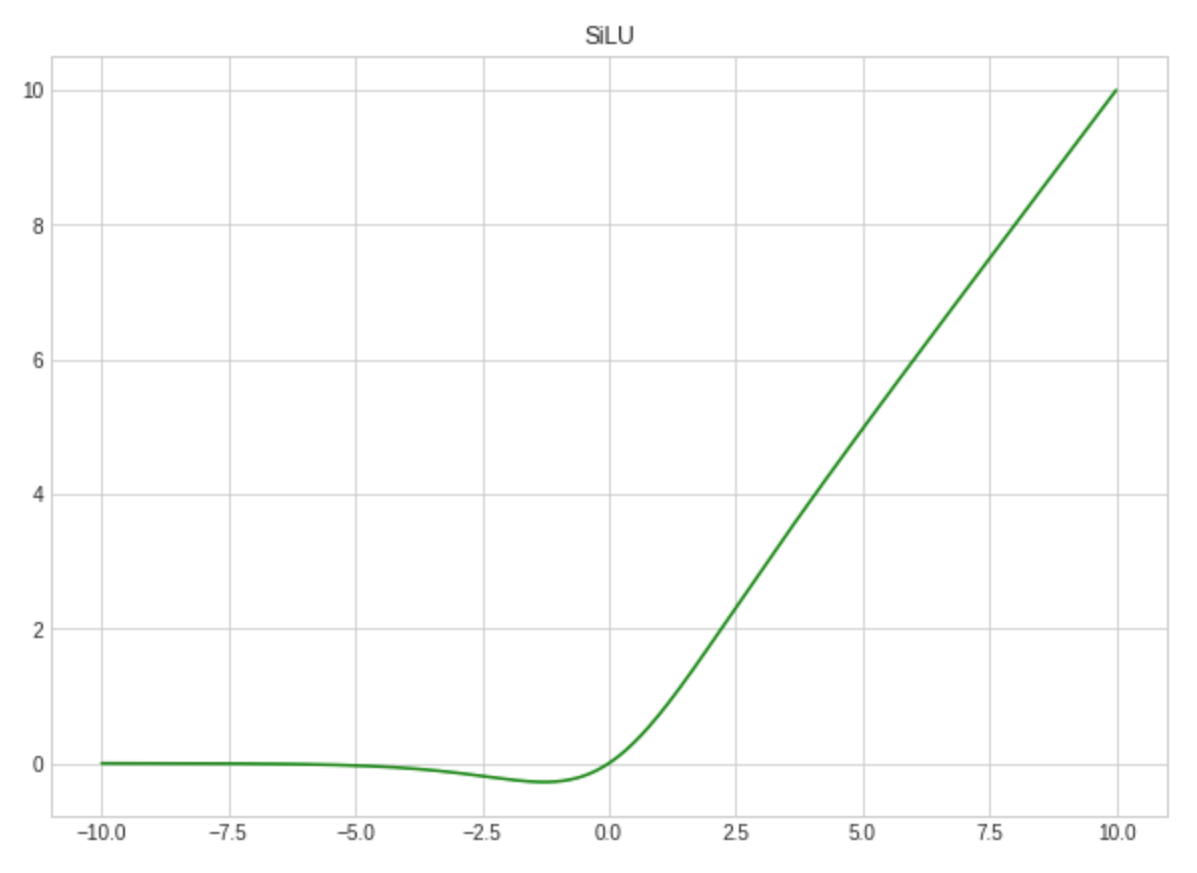SiLU

Such a simple activation function can be implemented just as easy as a Python function:

``````# simply define a silu function
def silu(input):
'''
Applies the Sigmoid Linear Unit (SiLU) function element-wise:

SiLU(x) = x * sigmoid(x)
'''
return input * torch.sigmoid(input) # use torch.sigmoid to make sure that we created the most efficient implemetation based on builtin PyTorch functions

# create a class wrapper from PyTorch nn.Module, so
# the function now can be easily used in models
class SiLU(nn.Module):
'''
Applies the Sigmoid Linear Unit (SiLU) function element-wise:

SiLU(x) = x * sigmoid(x)

Shape:
- Input: (N, *) where * means, any number of additional
dimensions
- Output: (N, *), same shape as the input

References:
-  Related paper:
https://arxiv.org/pdf/1606.08415.pdf

Examples:
>>> m = silu()
>>> input = torch.randn(2)
>>> output = m(input)

'''
def __init__(self):
'''
Init method.
'''
super().__init__() # init the base class

def forward(self, input):
'''
Forward pass of the function.
'''
return silu(input) # simply apply already implemented SiLU

``````

So now SiLU can be used in models created with nn.Sequential:

``````# use SiLU with model created with Sequential

# initialize activation function
activation_function = SiLU()

# Initialize the model using nn.Sequential
model = nn.Sequential(OrderedDict([
('fc1', nn.Linear(784, 256)),
('activation1', activation_function), # use SiLU
('fc2', nn.Linear(256, 128)),
('bn2', nn.BatchNorm1d(num_features=128)),
('activation2', activation_function), # use SiLU
('dropout', nn.Dropout(0.3)),
('fc3', nn.Linear(128, 64)),
('bn3', nn.BatchNorm1d(num_features=64)),
('activation3', activation_function), # use SiLU
('logits', nn.Linear(64, 10)),
('logsoftmax', nn.LogSoftmax(dim=1))]))

# Run training
train_model(model)

``````

Or in a simple model, which extends nn.Module class:

``````# create class for basic fully-connected deep neural network
class ClassifierSiLU(nn.Module):
'''
Demo classifier model class to demonstrate SiLU
'''
def __init__(self):
super().__init__()

# initialize layers
self.fc1 = nn.Linear(784, 256)
self.fc2 = nn.Linear(256, 128)
self.fc3 = nn.Linear(128, 64)
self.fc4 = nn.Linear(64, 10)

def forward(self, x):
# make sure the input tensor is flattened
x = x.view(x.shape, -1)

# apply silu function
x = silu(self.fc1(x))

# apply silu function
x = silu(self.fc2(x))

# apply silu function
x = silu(self.fc3(x))

x = F.log_softmax(self.fc4(x), dim=1)

return x

# Create demo model
model = ClassifierSiLU()

# Run training
train_model(model)

``````

## Implementing Activation Function with Trainable Parameters

There are lots of activation functions with parameters, which can be trained with gradient descent while training the model. A great example for one of these is Soft Exponential function: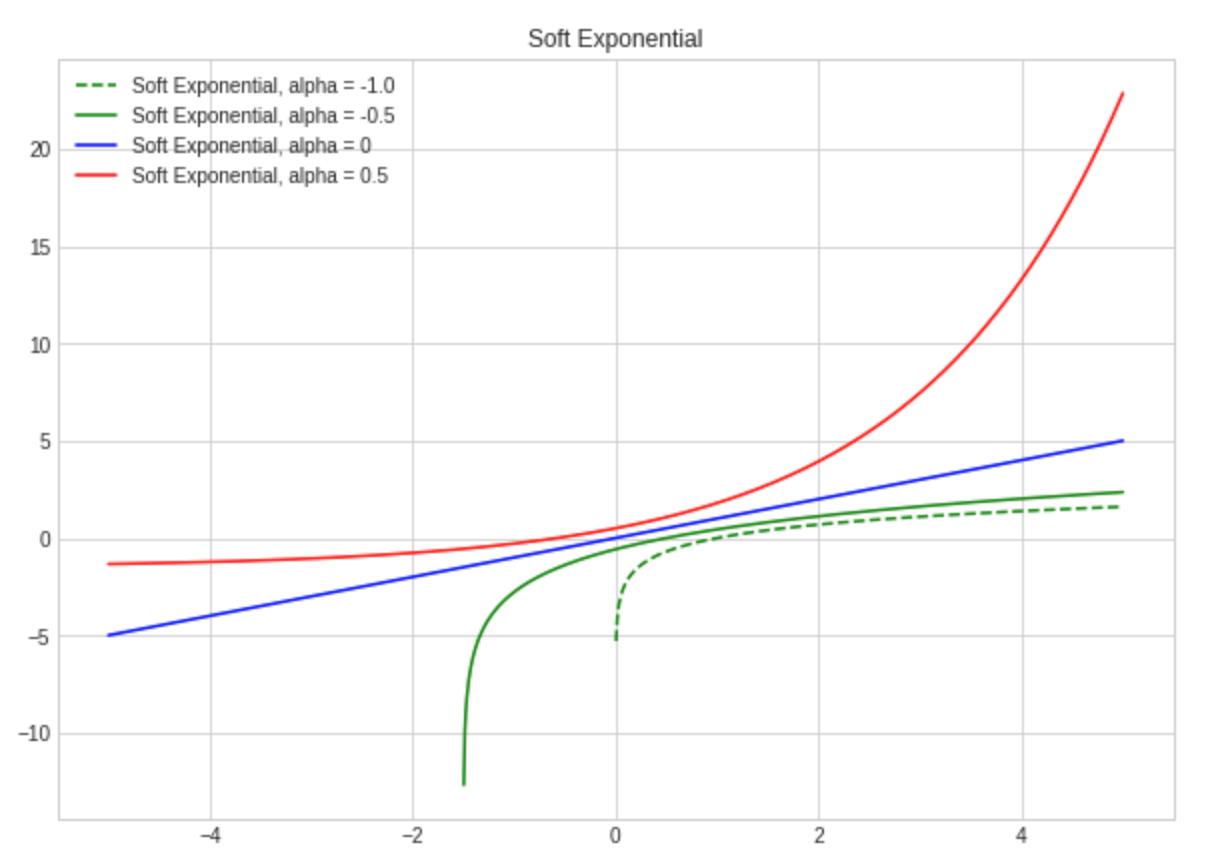Soft Exponential

To implement an activation function with trainable parameters we have to:

• derive a class from nn.Module and make the parameter one of its members,
• wrap the parameter as a PyTorch Parameter and set requiresGrad attribute to True.

Here is an example for Soft Exponential:

``````class soft_exponential(nn.Module):
'''
Implementation of soft exponential activation.

Shape:
- Input: (N, *) where * means, any number of additional
dimensions
- Output: (N, *), same shape as the input

Parameters:
- alpha - trainable parameter

References:
- See related paper:
https://arxiv.org/pdf/1602.01321.pdf

Examples:
>>> a1 = soft_exponential(256)
>>> x = torch.randn(256)
>>> x = a1(x)
'''
def __init__(self, in_features, alpha = None):
'''
Initialization.
INPUT:
- in_features: shape of the input
- aplha: trainable parameter
aplha is initialized with zero value by default
'''
super(soft_exponential,self).__init__()
self.in_features = in_features

# initialize alpha
if alpha == None:
self.alpha = Parameter(torch.tensor(0.0)) # create a tensor out of alpha
else:
self.alpha = Parameter(torch.tensor(alpha)) # create a tensor out of alpha

def forward(self, x):
'''
Forward pass of the function.
Applies the function to the input elementwise.
'''
if (self.alpha == 0.0):
return x

if (self.alpha < 0.0):
return - torch.log(1 - self.alpha * (x + self.alpha)) / self.alpha

if (self.alpha > 0.0):
return (torch.exp(self.alpha * x) - 1)/ self.alpha + self.alpha

``````

And now we can use Soft Exponential in our models as follows:

``````# create class for basic fully-connected deep neural network
class ClassifierSExp(nn.Module):
'''
Basic fully-connected network to test Soft Exponential activation.
'''
def __init__(self):
super().__init__()

# initialize layers
self.fc1 = nn.Linear(784, 256)
self.fc2 = nn.Linear(256, 128)
self.fc3 = nn.Linear(128, 64)
self.fc4 = nn.Linear(64, 10)

# initialize Soft Exponential activation
self.a1 = soft_exponential(256)
self.a2 = soft_exponential(128)
self.a3 = soft_exponential(64)

def forward(self, x):
# make sure the input tensor is flattened
x = x.view(x.shape, -1)

# apply Soft Exponential unit
x = self.a1(self.fc1(x))
x = self.a2(self.fc2(x))
x = self.a3(self.fc3(x))
x = F.log_softmax(self.fc4(x), dim=1)

return x

model = ClassifierSExp()
train_model(model)

``````

## Implementing Activation Function with Custom Backward Step

The perfect example of an activation function, which needs implementation of a custom backward step is BReLU (Bipolar Rectified Linear Unit):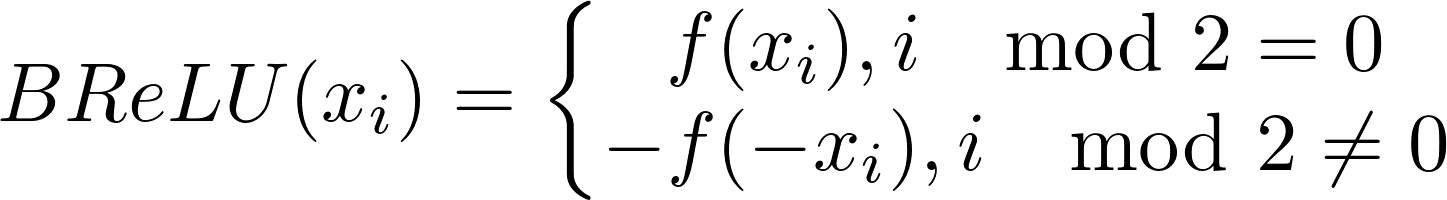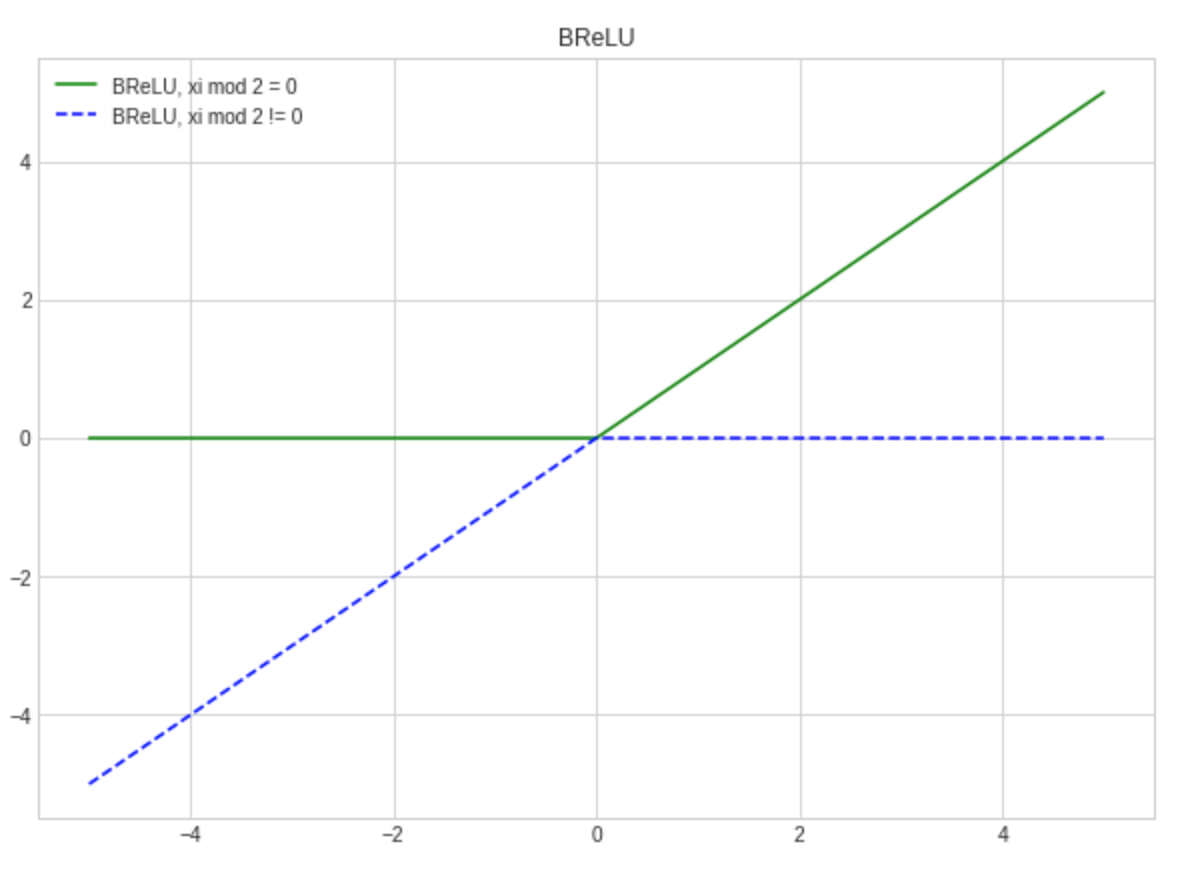BReLU

This function is not differentiable at 0, so automatic gradient computation might fail. That’s why we should provide a custom backward step to ensure stable computation.

To impement custom activation function with backward step we should:

• create a class which, inherits Function from torch.autograd,
• override static forward and backward methods. Forward method just applies the function to the input. Backward method computes the gradient of the loss function with respect to the input given the gradient of the loss function with respect to the output.

Let’s see an example for BReLU:

``````class brelu(Function):
'''
Implementation of BReLU activation function.

Shape:
- Input: (N, *) where * means, any number of additional
dimensions
- Output: (N, *), same shape as the input

References:
- See BReLU paper:
https://arxiv.org/pdf/1709.04054.pdf

Examples:
>>> brelu_activation = brelu.apply
>>> t = torch.randn((5,5), dtype=torch.float, requires_grad = True)
>>> t = brelu_activation(t)
'''
#both forward and backward are @staticmethods
@staticmethod
def forward(ctx, input):
"""
In the forward pass we receive a Tensor containing the input and return
a Tensor containing the output. ctx is a context object that can be used
to stash information for backward computation. You can cache arbitrary
objects for use in the backward pass using the ctx.save_for_backward method.
"""
ctx.save_for_backward(input) # save input for backward pass

# get lists of odd and even indices
input_shape = input.shape
even_indices = [i for i in range(0, input_shape, 2)]
odd_indices = [i for i in range(1, input_shape, 2)]

# clone the input tensor
output = input.clone()

# apply ReLU to elements where i mod 2 == 0
output[even_indices] = output[even_indices].clamp(min=0)

# apply inversed ReLU to inversed elements where i mod 2 != 0
output[odd_indices] = 0 - output[odd_indices] # reverse elements with odd indices
output[odd_indices] = - output[odd_indices].clamp(min = 0) # apply reversed ReLU

return output

@staticmethod
"""
In the backward pass we receive a Tensor containing the gradient of the loss
with respect to the output, and we need to compute the gradient of the loss
with respect to the input.
"""
grad_input = None # set output to None

input, = ctx.saved_tensors # restore input from context

# check that input requires grad
# if not requires grad we will return None to speed up computation

# get lists of odd and even indices
input_shape = input.shape
even_indices = [i for i in range(0, input_shape, 2)]
odd_indices = [i for i in range(1, input_shape, 2)]

``````

We can now use *BReLU *in our models as follows:

``````class ClassifierBReLU(nn.Module):
'''
Simple fully-connected classifier model to demonstrate BReLU activation.
'''
def __init__(self):
super(ClassifierBReLU, self).__init__()

# initialize layers
self.fc1 = nn.Linear(784, 256)
self.fc2 = nn.Linear(256, 128)
self.fc3 = nn.Linear(128, 64)
self.fc4 = nn.Linear(64, 10)

# create shortcuts for BReLU
self.a1 = brelu.apply
self.a2 = brelu.apply
self.a3 = brelu.apply

def forward(self, x):
# make sure the input tensor is flattened
x = x.view(x.shape, -1)

# apply BReLU
x = self.a1(self.fc1(x))
x = self.a2(self.fc2(x))
x = self.a3(self.fc3(x))
x = F.log_softmax(self.fc4(x), dim=1)

return x

model = ClassifierBReLU()
train_model(model)

``````

## Conclusion

In this tutorial I covered:

• How to create a simple custom activation function with PyTorch,
• How to create an** activation function with trainable parameters**, which can be trained using gradient descent,
• How to create an activation function with a custom backward step.

All code from this tutorial is available on GitHub. Other examples of implemented custom activation functions for PyTorch and Keras you can find in this GitHub repository.

#python #deep-learning #machine-learning

## Buddha Community1599097440

## Data Visualization in R with ggplot2: A Beginner Tutorial

A famous general is thought to have said, “A good sketch is better than a long speech.” That advice may have come from the battlefield, but it’s applicable in lots of other areas — including data science. “Sketching” out our data by visualizing it using ggplot2 in R is more impactful than simply describing the trends we find.

This is why we visualize data. We visualize data because it’s easier to learn from something that we can see rather than read. And thankfully for data analysts and data scientists who use R, there’s a tidyverse package called ggplot2 that makes data visualization a snap!

In this blog post, we’ll learn how to take some data and produce a visualization using R. To work through it, it’s best if you already have an understanding of R programming syntax, but you don’t need to be an expert or have any prior experience working with ggplot2

#data science tutorials #beginner #ggplot2 #r #r tutorial #r tutorials #rstats #tutorial #tutorials1596728880

## Tutorial: Getting Started with R and RStudio

In this tutorial we’ll learn how to begin programming with R using RStudio. We’ll install R, and RStudio RStudio, an extremely popular development environment for R. We’ll learn the key RStudio features in order to start programming in R on our own.

If you already know how to use RStudio and want to learn some tips, tricks, and shortcuts, check out this Dataquest blog post.

#data science tutorials #beginner #r tutorial #r tutorials #rstats #tutorial #tutorials1596513720

## 1. Characteristics of Clean Data and Messy Data

What exactly is clean data? Clean data is accurate, complete, and in a format that is ready to analyze. Characteristics of clean data include data that are:

• Free of duplicate rows/values
• Error-free (e.g. free of misspellings)
• Relevant (e.g. free of special characters)
• The appropriate data type for analysis
• Free of outliers (or only contain outliers have been identified/understood), and
• Follows a “tidy data” structure

Common symptoms of messy data include data that contain:

• Special characters (e.g. commas in numeric values)
• Numeric values stored as text/character data types
• Duplicate rows
• Misspellings
• Inaccuracies
• White space
• Missing data
• Zeros instead of null values

## 2. Motivation

In this blog post, we will work with five property-sales datasets that are publicly available on the New York City Department of Finance Rolling Sales Data website. We encourage you to download the datasets and follow along! Each file contains one year of real estate sales data for one of New York City’s five boroughs. We will work with the following Microsoft Excel files:

• rollingsales_bronx.xls
• rollingsales_brooklyn.xls
• rollingsales_manhattan.xls
• rollingsales_queens.xls
• rollingsales_statenisland.xls

As we work through this blog post, imagine that you are helping a friend launch their home-inspection business in New York City. You offer to help them by analyzing the data to better understand the real-estate market. But you realize that before you can analyze the data in R, you will need to diagnose and clean it first. And before you can diagnose the data, you will need to load it into R!

Benefits of using tidyverse tools are often evident in the data-loading process. In many cases, the tidyverse package `readxl` will clean some data for you as Microsoft Excel data is loaded into R. If you are working with CSV data, the tidyverse `readr` package function `read_csv()` is the function to use (we’ll cover that later).

Let’s look at an example. Here’s how the Excel file for the Brooklyn borough looks: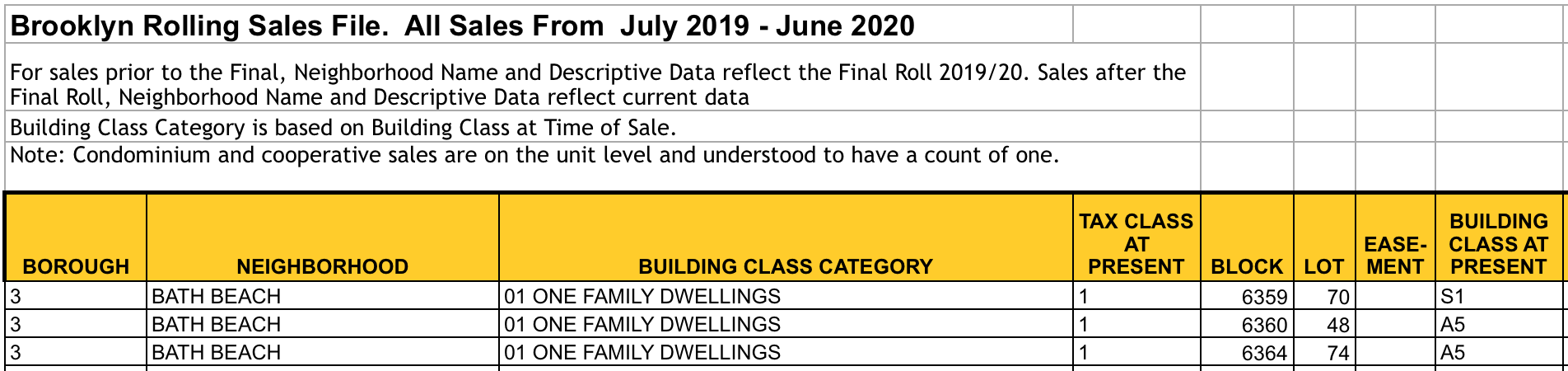The Brooklyn Excel file

Now let’s load the Brooklyn dataset into R from an Excel file. We’ll use the `readxl`package. We specify the function argument `skip = 4` because the row that we want to use as the header (i.e. column names) is actually row 5. We can ignore the first four rows entirely and load the data into R beginning at row 5. Here’s the code:

``````library(readxl) # Load Excel files
brooklyn <- read_excel("rollingsales_brooklyn.xls", skip = 4)
``````

Note we saved this dataset with the variable name `brooklyn` for future use.

## 4. View the Data with tidyr::glimpse()

The tidyverse offers a user-friendly way to view this data with the `glimpse()` function that is part of the `tibble` package. To use this package, we will need to load it for use in our current session. But rather than loading this package alone, we can load many of the tidyverse packages at one time. If you do not have the tidyverse collection of packages, install it on your machine using the following command in your R or R Studio session:

``````install.packages("tidyverse")
``````

Once the package is installed, load it to memory:

``````library(tidyverse)
``````

Now that `tidyverse` is loaded into memory, take a “glimpse” of the Brooklyn dataset:

``````glimpse(brooklyn)
## Observations: 20,185
## Variables: 21
## \$ BOROUGH <chr> "3", "3", "3", "3", "3", "3", "…
## \$ NEIGHBORHOOD <chr> "BATH BEACH", "BATH BEACH", "BA…
## \$ `BUILDING CLASS CATEGORY` <chr> "01 ONE FAMILY DWELLINGS", "01 …
## \$ `TAX CLASS AT PRESENT` <chr> "1", "1", "1", "1", "1", "1", "…
## \$ BLOCK <dbl> 6359, 6360, 6364, 6367, 6371, 6…
## \$ LOT <dbl> 70, 48, 74, 24, 19, 32, 65, 20,…
## \$ `EASE-MENT` <lgl> NA, NA, NA, NA, NA, NA, NA, NA,…
## \$ `BUILDING CLASS AT PRESENT` <chr> "S1", "A5", "A5", "A9", "A9", "…
## \$ ADDRESS <chr> "8684 15TH AVENUE", "14 BAY 10T…
## \$ `APARTMENT NUMBER` <chr> NA, NA, NA, NA, NA, NA, NA, NA,…
## \$ `ZIP CODE` <dbl> 11228, 11228, 11214, 11214, 112…
## \$ `RESIDENTIAL UNITS` <dbl> 1, 1, 1, 1, 1, 1, 1, 1, 2, 1, 1…
## \$ `COMMERCIAL UNITS` <dbl> 1, 0, 0, 0, 0, 0, 0, 0, 0, 0, 0…
## \$ `TOTAL UNITS` <dbl> 2, 1, 1, 1, 1, 1, 1, 1, 2, 1, 1…
## \$ `LAND SQUARE FEET` <dbl> 1933, 2513, 2492, 1571, 2320, 3…
## \$ `GROSS SQUARE FEET` <dbl> 4080, 1428, 972, 1456, 1566, 22…
## \$ `YEAR BUILT` <dbl> 1930, 1930, 1950, 1935, 1930, 1…
## \$ `TAX CLASS AT TIME OF SALE` <chr> "1", "1", "1", "1", "1", "1", "…
## \$ `BUILDING CLASS AT TIME OF SALE` <chr> "S1", "A5", "A5", "A9", "A9", "…
## \$ `SALE PRICE` <dbl> 1300000, 849000, 0, 830000, 0, …
## \$ `SALE DATE` <dttm> 2020-04-28, 2020-03-18, 2019-0…
``````

The `glimpse()` function provides a user-friendly way to view the column names and data types for all columns, or variables, in the data frame. With this function, we are also able to view the first few observations in the data frame. This data frame has 20,185 observations, or property sales records. And there are 21 variables, or columns.

#data science tutorials #beginner #r #r tutorial #r tutorials #rstats #tidyverse #tutorial #tutorials1594399440

## Getting Started with R Markdown — Guide and Cheatsheet

In this blog post, we’ll look at how to use R Markdown. By the end, you’ll have the skills you need to produce a document or presentation using R Mardown, from scratch!

We’ll show you how to convert the default R Markdown document into a useful reference guide of your own. We encourage you to follow along by building out your own R Markdown guide, but if you prefer to just read along, that works, too!

R Markdown is an open-source tool for producing reproducible reports in R. It enables you to keep all of your code, results, plots, and writing in one place. R Markdown is particularly useful when you are producing a document for an audience that is interested in the results from your analysis, but not your code.

R Markdown is powerful because it can be used for data analysis and data science, collaborating with others, and communicating results to decision makers. With R Markdown, you have the option to export your work to numerous formats including PDF, Microsoft Word, a slideshow, or an HTML document for use in a website.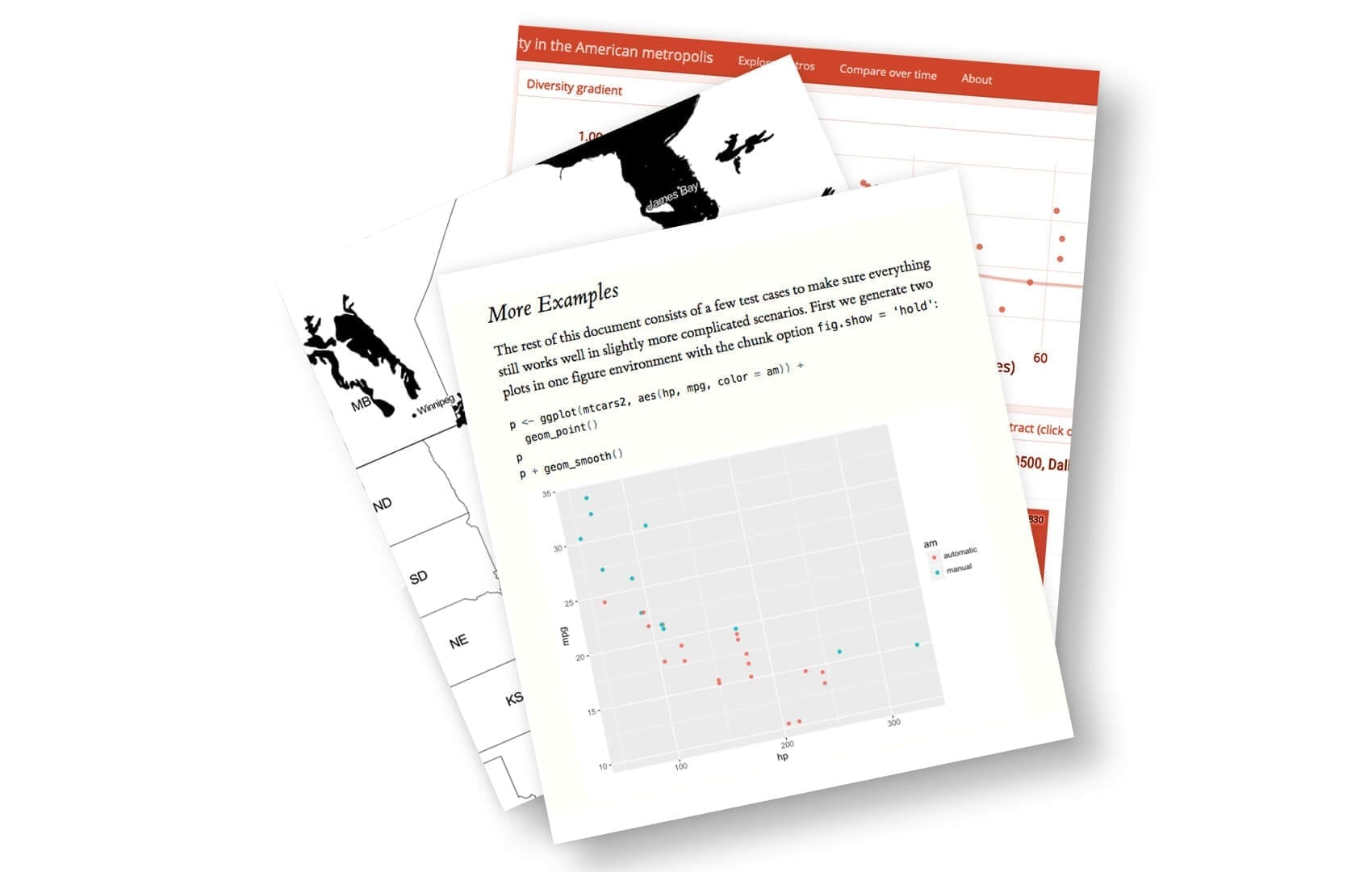Turn your data analysis into pretty documents with R Markdown.

We’ll use the RStudio integrated development environment (IDE) to produce our R Markdown reference guide. If you’d like to learn more about RStudio, check out our list of 23 awesome RStudio tips and tricks!

Here at Dataquest, we love using R Markdown for coding in R and authoring content. In fact, we wrote this blog post in R Markdown! Also, learners on the Dataquest platform use R Markdown for completing their R projects.

We included fully-reproducible code examples in this blog post. When you’ve mastered the content in this post, check out our other blog post on R Markdown tips, tricks, and shortcuts.

Okay, let’s get started with building our very own R Markdown reference document!

## 1. Install R Markdown

R Markdown is a free, open source tool that is installed like any other R package. Use the following command to install R Markdown:

``````install.packages("rmarkdown")
``````

Now that R Markdown is installed, open a new R Markdown file in RStudio by navigating to `File > New File > R Markdown…`. R Markdown files have the file extension “.Rmd”.

## 2. Default Output Format

When you open a new R Markdown file in RStudio, a pop-up window appears that prompts you to select output format to use for the document.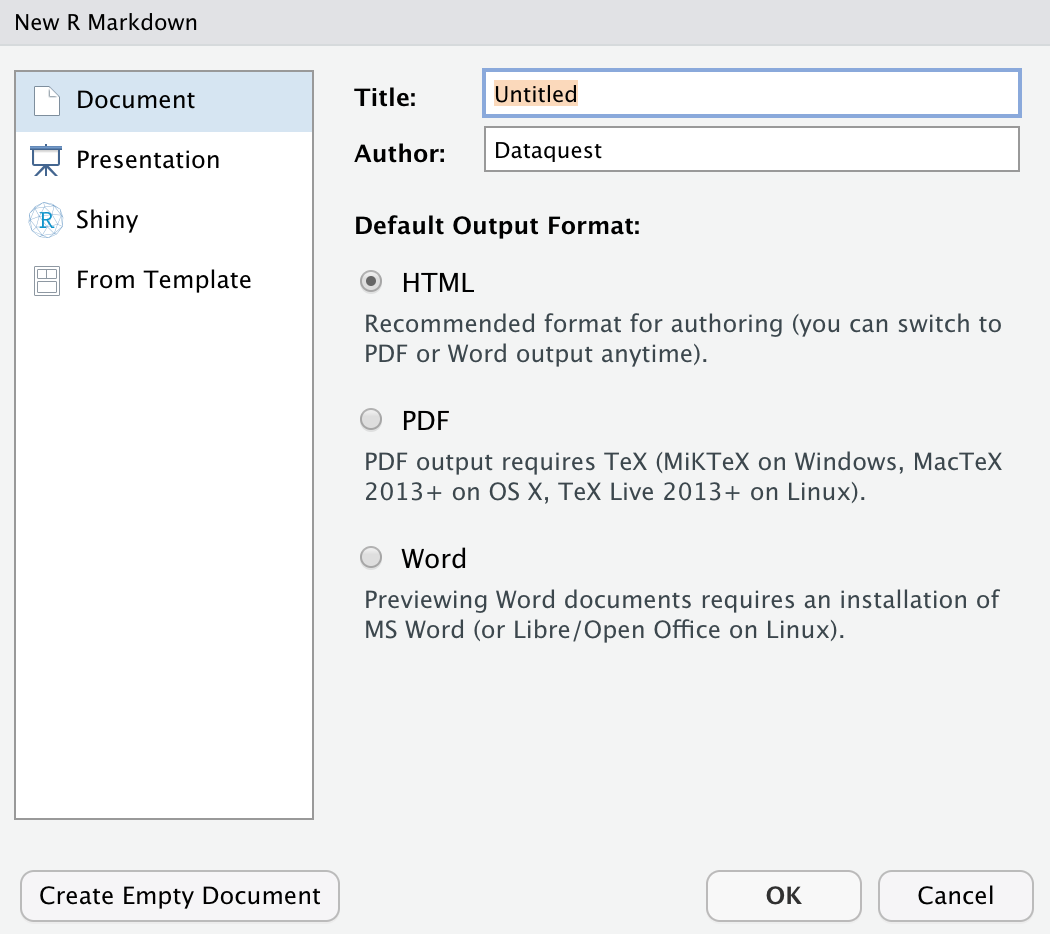The default output format is HTML. With HTML, you can easily view it in a web browser.

We recommend selecting the default HTML setting for now — it can save you time! Why? Because compiling an HTML document is generally faster than generating a PDF or other format. When you near a finished product, you change the output to the format of your choosing and then make the final touches.

One final thing to note is that the title you give your document in the pop-up above is not the file name! Navigate to `File > Save As..` to name, and save, the document.

#data science tutorials #beginner #r #r markdown #r tutorial #r tutorials #rstats #rstudio #tutorial #tutorials

1617089618

## Laravel 8 Tutorial for Beginners

Hello everyone! I just updated this tutorial for Laravel 8. In this tutorial, we’ll go through the basics of the Laravel framework by building a simple blogging system. Note that this tutorial is only for beginners who are interested in web development but don’t know where to start. Check it out if you are interested: Laravel Tutorial For Beginners

Laravel is a very powerful framework that follows the MVC structure. It is designed for web developers who need a simple, elegant yet powerful toolkit to build a fully-featured website.

Recommended:-Laravel Try Catch

#laravel 8 tutorial #laravel 8 tutorial crud #laravel 8 tutorial point #laravel 8 auth tutorial #laravel 8 project example #laravel 8 tutorial for beginners